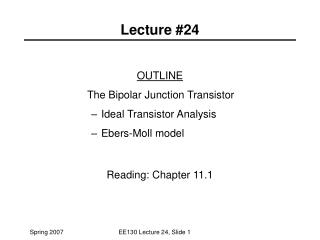DownloadDownload PresentationLecture #24

# Lecture #24

Télécharger la présentation## Lecture #24

- - - - - - - - - - - - - - - - - - - - - - - - - - - E N D - - - - - - - - - - - - - - - - - - - - - - - - - - -
##### Presentation Transcript

1. OUTLINE The Bipolar Junction Transistor Ideal Transistor Analysis Ebers-Moll model Reading: Chapter 11.1 Lecture #24 EE130 Lecture 24, Slide 1

2. Diffusion equation: General solution: Boundary conditions: Solution: Emitter Region Solution EE130 Lecture 24, Slide 2

3. Collector Region Solution • Diffusion equation: • General solution: • Boundary conditions: • Solution: EE130 Lecture 24, Slide 3

4. Base Region Solution • Diffusion equation: • General solution: • Boundary conditions: • Solution: EE130 Lecture 24, Slide 4

5. Since we can write as EE130 Lecture 24, Slide 5

6. EE130 Lecture 24, Slide 6

7. We know: Therefore: Terminal Currents EE130 Lecture 24, Slide 7

8. In real BJTs, we make W << LB to achieve high current gain. Then, since we have: Simplification EE130 Lecture 24, Slide 8

9. BJT Performance Parameters • Assumptions: • emitter junction forward biased, collector junction reverse biased • W << LB EE130 Lecture 24, Slide 9

10. BJT with Narrow Emitter Replace with WE’ if short emitter EE130 Lecture 24, Slide 10

11. Ebers-Moll Model increasing The Ebers-Moll model is a large-signal equivalent circuit which describes both the active and saturation regions of BJT operation. EE130 Lecture 24, Slide 11

12. V V EB CB I B E B C I C If only VEB is applied (VCB = 0): aR : reverse common base gain aF : forward common base gain If only VCBis applied (VEB = 0): : Reciprocity relationship: EE130 Lecture 24, Slide 12

13. In the general case, both VEB and VCB are non-zero: IC: C-B diode current + fraction of E-B diode current that makes it to the C-B junction IE: E-B diode current + fraction of C-B diode current that makes it to the E-B junction Large-signal equivalent circuit for a pnp BJT EE130 Lecture 24, Slide 13